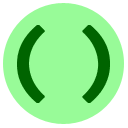# 13: 序列 (数组)

`myList = ["the first value in the list", 1999, 4.5]`

`«listName»[«indexNumber»]````stuff = [2, 25, 80, 12]
stuff[stuff] = stuff
print(stuff)```Python里面的序列在其他编程语言里面被叫做数组。Python 里有一些不同且更加高级的东西&，也被称作数组。

### 序列的长度: `len(«list»)`### 串联与创造### 行末：负指数

`«listName»[-1]``racecar`

### `max`和`sum`### 循环序列

```for x in L:
«loop body block»```"For all"循环也适用于字符串：尝试`for char in "hello"`.`x`为何值时，会使`mystery(x)`一直运行下去？

```def mystery(x):
a = [0, 4, 0, 3, 2]
while x > 0:
x = a[x]
return "Done"```• for i in range(0, 10):
• def mode(L):
• frequency = *10
• frequency[i] = frequency[i] + 1
• if frequency[i]==max(frequency):
• for i in L:
• return i